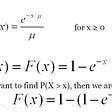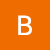# Dijkstra’s Shortest Path Algorithm

Dijkstra’s shortest path algorithm is one algorithm of many that can be used to find the shortest path between two nodes of a graph. However, what makes the algorithm unique is the fact that it generates a table that can be used to determine the shortest path between not only two specific nodes, but any two nodes that have an existing path between them within the graph. Because it is a greedy algorithm, Dijkstra’s algorithm may not always be the most optimal solution to finding the shortest path between two nodes, but it makes up for these deficiencies with other benefits such as the fact that the algorithm only needs to be run once to determine the shortest between any two nodes in the graph as long as the graph remains unchanged.

# An overview of the algorithm

A basic overview of the algorithm is as follows.

1. We begin at the starting node and visit all of its adjacent nodes while keeping track of the distance from the initial node to adjacent nodes. If the distance is smaller than the previous known shortest distance, we update the distance with the smaller distance and mark the previous vertex.
2. After all neighboring nodes have been visited, we will mark the starting node as visited. Visited nodes will not be visited again.
3. We then choose an unvisited vertex with the smallest known distance from the starting node.
4. Visit all of its unvisited neighbors and note their distances from the starting node. If the distance is smaller than current known shortest distances from the starting node, update the table with the smaller distance and the previous vertex, that being the current node.
5. Repeat these steps until all unvisited nodes have been visited.

# Visualizing The Algorithm

Let’s draw out the algorithm so we can visualize how we could find the shortest path from node A to node C in the graph below.

First, we begin by initializing the table we will be using to keep track of information while we perform each step of the algorithm. The starting node will have a distance of 0 because we know that the distance from the starting node to itself is 0. All other distances are currently unknown, and therefore, we’ll set them all as infinity. We must also keep track of visited and unvisited nodes so we’ll keep track of a list of both. Initially, the visited node list will be empty as we haven’t visited any nodes and the unvisited node list will contain all nodes in the graph.

Next, we choose the next unvisited node with the smallest distance from the starting node. This happens to be node A. We then check any unvisited neighbors of A which are B and D and update their distances if they are shorter than the current known distances. In this case, A to B has a distance of 6 and it’s current known distance is infinity, so we will update the distance of A to B to 6. Similarly, the current known distance of A to D is infinity, but we have now calculated the new distance to be 1, so infinity will be replaced by 1. Now that we have finished visiting all neighbors of A, we will move A from the unvisited list to the visited list.

We will now move on to the next unvisited node in the graph that has the smallest known distance from starting node A. Since A is now in the visited list, the next smallest option is node D. Node D’s unvisited neighbors are B and E. The distance from node D to node B is the distance of node A to node D plus the distance of node D to node B which is 3. Since the previous distance from the starting node to B was 6 and the newly calculated distance is 3, we will replace 6 with 3 and set node B’s previous vertex to our current vertex, D. Repeating the process for node D to node E, we find that the calculated distance is 1+1 which is less than the already known shortest distance of infinity. Therefore, we update the known distance to E from A to 2. Now that we have visited all of node D’s neighbors, we will move it to the visited list. Our resulting table will now look as it does below.

I will refrain from listing out every step before the table is completed as the subsequent steps should be more clear now. After stepping through the rest of the algorithm yourself, your table should look like this.

With the table complete, you are now able to find the shortest path from any two nodes in the graph and also the exact path! Let’s find the path and shortest distance from node A to node C. We can already see from the table that the shortest distance to node C from the starting node is 7. To find the path, we begin at node C and check it’s previous vertex which is E. We then find E’s previous vertex which is D. D’s previous vertex is A. Going backwards from there, you can see that the shortest pathfrom node A to node C is from A to D to E to C.

# Implementing the Algorithm With Code

Let’s list out the steps required to implement Dijkstra’s algorithm with code.

1. Build the table that will hold information about each vertex’s distance from the starting node and previous vertex. We will use a dictionary with the key being the current vertex.
2. Initialize two arrays. An array for unvisited nodes and an array for visited nodes.
3. Begin iterating through the dictionary at the starting vertex. Check if the neighbors of the current vertex have been visited.
4. If the neighbor hasn’t been visited, we keep track of the neighbor’s vertex name, distance to the neighbor, and current known cost to neighbor.
5. Compare distance to the neighbor with the current known cost to the neighbor. If the distance to the neighbor is less than the current known cost, update the current known cost to the calculated distance. If not, leave the current known cost as is.
6. After checking all neighbors of the current vertex, move the current vertex from the unvisited array to the visited array.
7. Iterate through the unvisited array and find the unvisited node with the lowest known distance. Set the found node to the current node and repeat.
8. Repeat the loop until the unvisited array is empty. Then return the table.

Now let’s see it with code!

`def build_shortest_path(graph, start):   #Create shortest path table   table = {}   for i in graph:      if i == start:         table[i] = [0, '']      else:         table[i] = [math.inf, '']   #Create visited and unvisited node arrays   visited, unvisited = [], [key for key in graph]   current = start   neighbors = graph[current]   #Continue until unvisited list is empty   while len(unvisited) != 0:      for n in neighbors:         if n not in visited:            name, neighbor_cost, current_cost = n, n, table[current]            distance = current_cost + neighbor_cost            cost_in_table = table[name]              if distance < cost_in_table:               table[name], table[name] = distance, current      visited.append(current)      unvisited.remove(current)      minimum = math.inf      for v in unvisited:         if table[v] < minimum:            minimum = table[v]      for v in unvisited:         if table[v] == minimum:            current = v            neighbors = graph[current]      return table`

The above function only returns the complete table. We must now use this table to find the shortest path itself, from start to end.

`def get_shortest_path(table, start, end):   #Initialize an array to store path   path = []   current = end   #Starting from the end, use the table to find each vertex's   #previous vertex until the indicated start is found   while start not in path:      path.append(current)      current = table[current]   #The current stored path will be backwards. Reverse it   path.reverse()   return path`

And there you have it! You now (hopefully) have a basic understanding of Dijkstra’s algorithm and its implementation in code.

--

--

--

## More from Ben Chan

Current CS student and aspiring software engineer

Love podcasts or audiobooks? Learn on the go with our new app.

## Recommended from Medium## The Perfect Matching## The geometric interpretation of 3D lines and planes## Depth first and breadth first search on trees in Javascript## Distributions## Herr Kretschmann attack on Einstein’s theory of General Relativity (1917)## Ben Chan

Current CS student and aspiring software engineer

## Substring Search using KMP (Knuth–Morris–Pratt) algorithm## Processing Medical Records